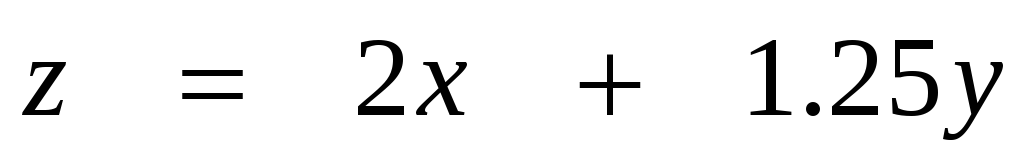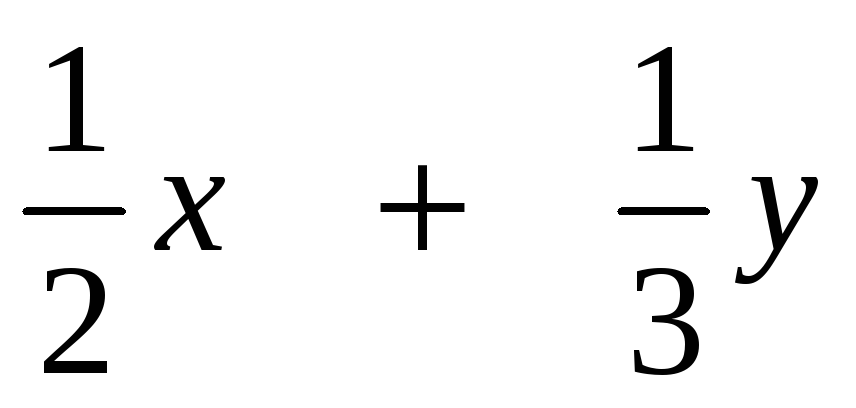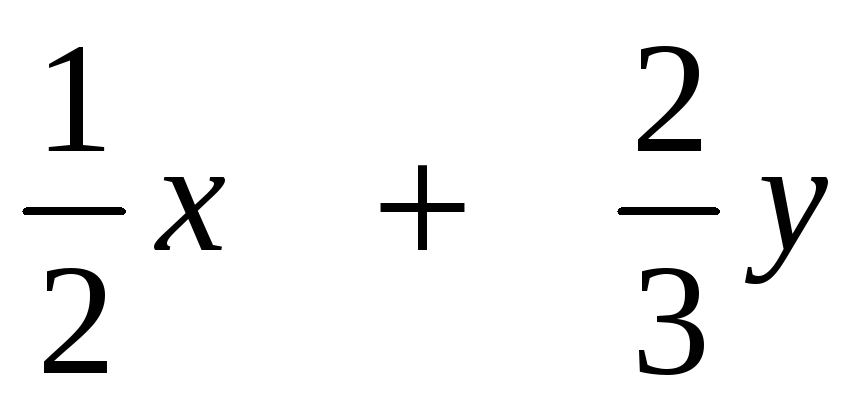# Linear Programming Formulation and Solution Example 1

 Linear Programming Formulation and Solution Example 1 A candy manufacturer has 130 pounds of chocolate-covered cherries and 170 pounds of chocolate-covered mints in stock. He decides to sell them in the form of two different mixtures. One mixture will contain half cherries and half mints by weight and will sell for \$2.00 per pound. The other mixture will contain one-third cherries and two-thirds mints by weight and will sell for \$1.25 per pound. How many pounds of each mixture should the candy manufacturer prepare in order to maximize his sales revenue?   Solution For simplicity, let us call A the mixture of half cherries and half mints, and B the mixture which is one-third cherries and two-thirds mints. Let x be the number of pounds of A to be prepared and y the number of pounds of B to be prepared. The revenue function can then be written asSince each pound of A contains one-half pound of cherries and each pound of B contains one-third pound of cherries, the total number of pounds of cherries used in both mixtures isSimilarly, the total number of pounds of mints used in both mixtures is:Share with your friends: